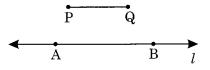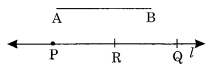# NCERT Solutions For Class 6 Maths Practical Geometry Exercise 14.3

ncert textbook

## NCERT Solutions For Class 6 Maths Practical Geometry Exercise 14.3

NCERT Solutions For Class 6 Maths Chapter 14 Practical Geometry Ex 14.3

Exercise 14.3

Ex 14.3 Class 6 Maths Question 1.
Draw any line segment$\overline { PQ }$. Without measuring$\overline { PQ }$, construct a copy of$\overline { PQ }$.
Solution:
Step I: Draw$\overline { PQ }$of unknown length.
Step II : Draw a line l and mark a point A on it.
Step III: Open the compass equal to PQ.
Step IV : Place the needle of the compass at A and mark a point B on l.
Thus,$\overline { AB }$is a copy of$\overline { PQ }$.Ex 14.3 Class 6 Maths Question 2.
Given some line segment$\overline { AB }$whose length you do not know, construct$\overline { PQ }$such that the length of$\overline { PQ }$is twice that of$\overline { AB }$.
Solution:
Step I : Draw$\overline { AB }$of any suitable length.
Step II : Place the needle of the compass at A and the other pencil end at B.
Step III : Draw a line l and take a point P on it.
Step IV: With the same opening of the compass, place the needle at P and mark another point Q on l.
Thus$\overline { PQ }$is the required line segment whose length is twice the length of$\overline { AB }$i.e$\overline { PQ }$=$\overline { 2AB }$.